Problem:

On an infinite number line (x-axis), we drop given squares in the order they are given.

The i-th square dropped (positions[i] = (left, side_length)) is a square with the left-most point being positions[i] and sidelength positions[i].

The square is dropped with the bottom edge parallel to the number line, and from a higher height than all currently landed squares. We wait for each square to stick before dropping the next.

The squares are infinitely sticky on their bottom edge, and will remain fixed to any positive length surface they touch (either the number line or another square). Squares dropped adjacent to each other will not stick together prematurely.

Return a list ans of heights. Each height ans[i] represents the current highest height of any square we have dropped, after dropping squares represented by positions, positions, ..., positions[i].

Example 1:

Example 2:

Note:

• 1 <= positions.length <= 1000.
• 1 <= positions[i] <= 10^8.
• 1 <= positions[i] <= 10^6.

Idea:

Range query with map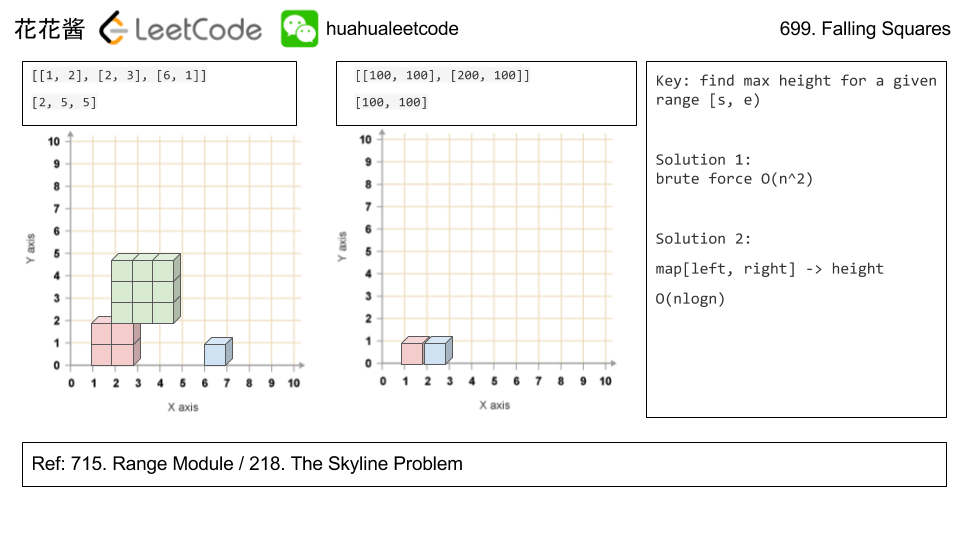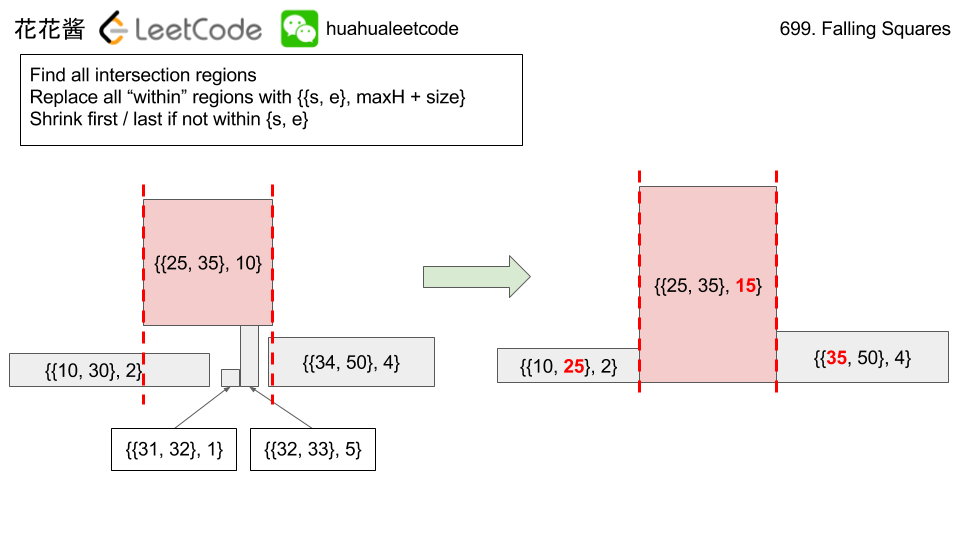Solution:

C++ map

C++ vector without merge

C++ / vector with merge (slower)

Related Problems:

Problem:

A Range Module is a module that tracks ranges of numbers. Your task is to design and implement the following interfaces in an efficient manner.

• addRange(int left, int right) Adds the half-open interval [left, right), tracking every real number in that interval. Adding an interval that partially overlaps with currently tracked numbers should add any numbers in the interval [left, right) that are not already tracked.
• queryRange(int left, int right) Returns true if and only if every real number in the interval [left, right) is currently being tracked.
• removeRange(int left, int right) Stops tracking every real number currently being tracked in the interval [left, right).

Example 1:

Note:

• A half open interval [left, right) denotes all real numbers left <= x < right.
• 0 < left < right < 10^9 in all calls to addRange, queryRange, removeRange.
• The total number of calls to addRange in a single test case is at most 1000.
• The total number of calls to queryRange in a single test case is at most 5000.
• The total number of calls to removeRange in a single test case is at most 1000.

Idea:

map / ordered ranges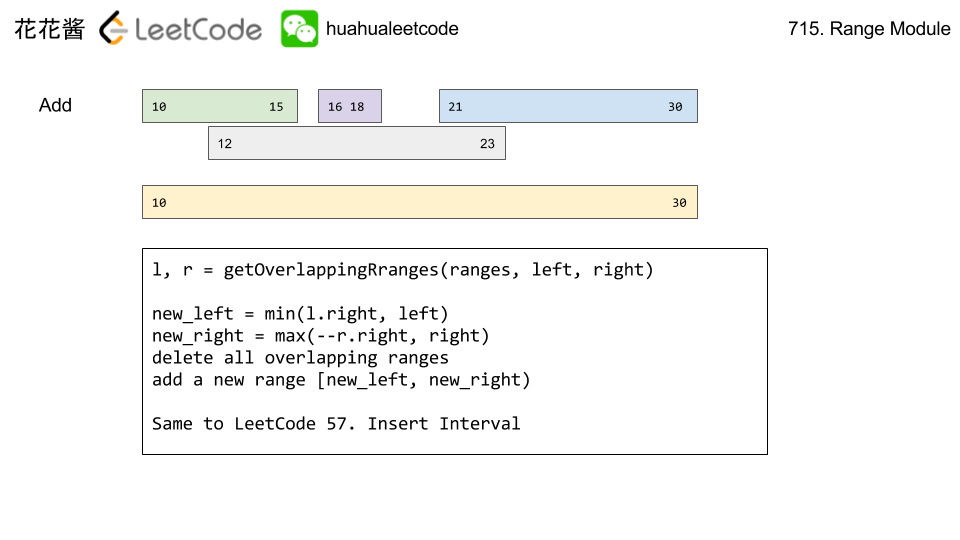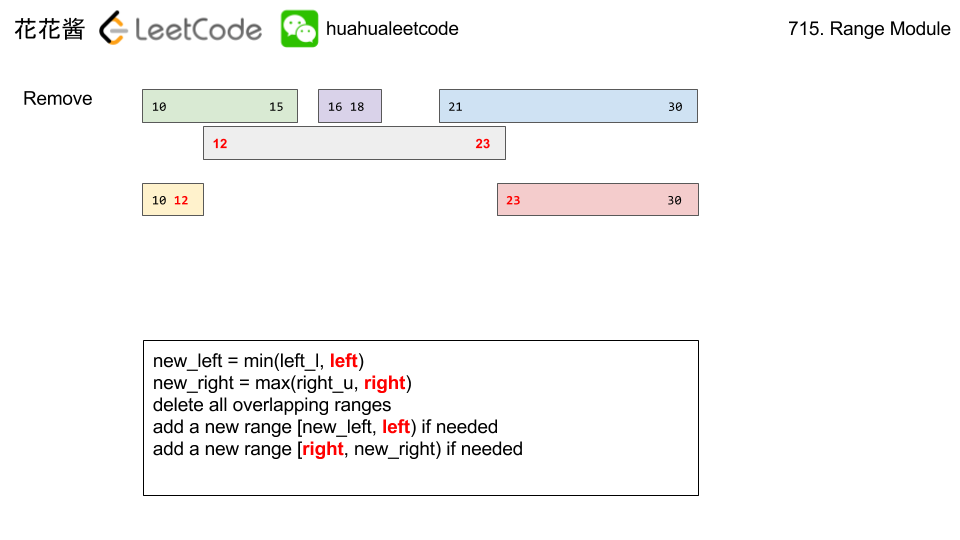Solution:

C++ / vector

C++ / map

Related Problems:

Problem:

Given a non-empty array of integers, return the k most frequent elements.

For example,
Given [1,1,1,2,2,3] and k = 2, return [1,2].

Note:
You may assume k is always valid, 1 ≤ k ≤ number of unique elements.
Your algorithm’s time complexity must be better than O(n log n), where n is the array’s size.

Idea: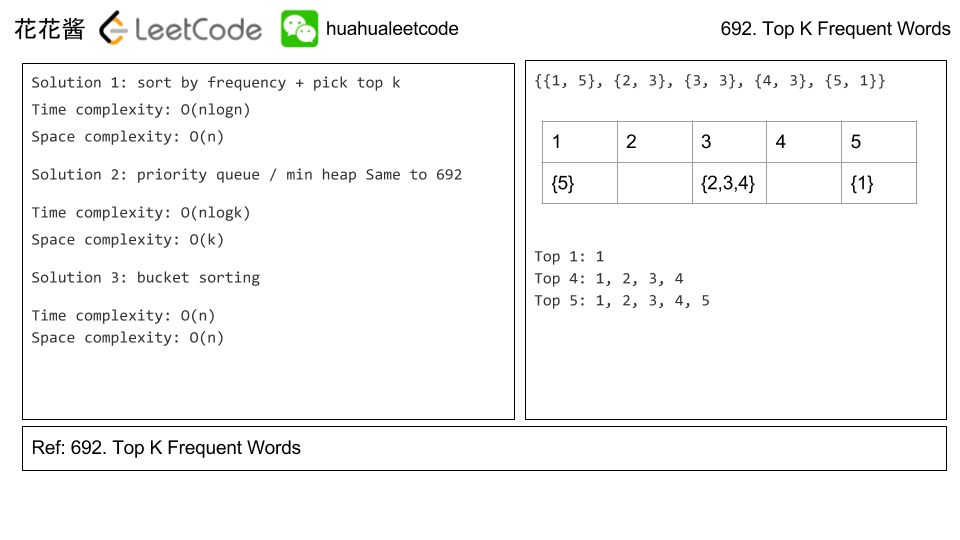# Solution 2: Priority queue / max heap

Time complexity: O(n) + O(nlogk)

Space complexity: O(n)

# Solution 3: Bucket Sort

Time complexity: O(n)

Space complexity: O(n)

# Related Problems

Problem:

Given a non-empty list of words, return the k most frequent elements.

Your answer should be sorted by frequency from highest to lowest. If two words have the same frequency, then the word with the lower alphabetical order comes first.

Example 1:

Example 2:

Note:

1. You may assume k is always valid, 1 ≤ k ≤ number of unique elements.
2. Input words contain only lowercase letters.

1. Try to solve it in O(n log k) time and O(n) extra space.

Idea:

Priority queue / min heap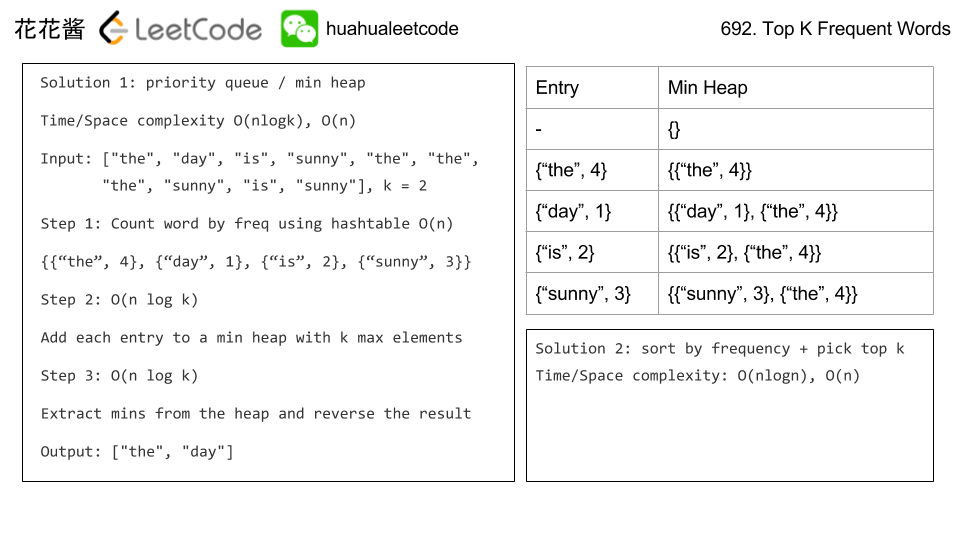Solution

C++ / priority_queue O(n log k) / O(n)

Related Problems

Problem:

There are a total of n courses you have to take, labeled from 0 to n - 1.

Some courses may have prerequisites, for example to take course 0 you have to first take course 1, which is expressed as a pair: [0,1]

Given the total number of courses and a list of prerequisite pairs, is it possible for you to finish all courses?

For example:

There are a total of 2 courses to take. To take course 1 you should have finished course 0. So it is possible.

There are a total of 2 courses to take. To take course 1 you should have finished course 0, and to take course 0 you should also have finished course 1. So it is impossible.

Idea:

Finding cycles O(n^2) -> Topological sort O(n)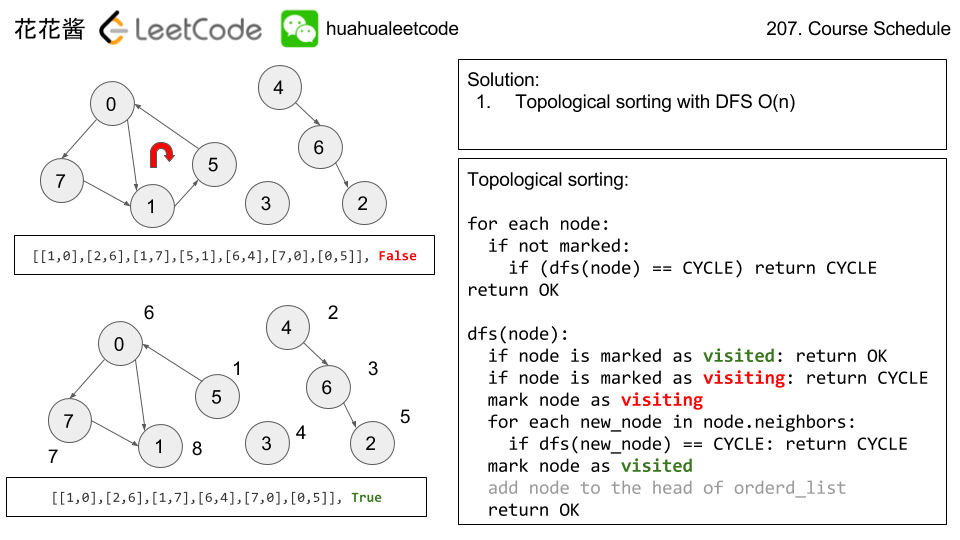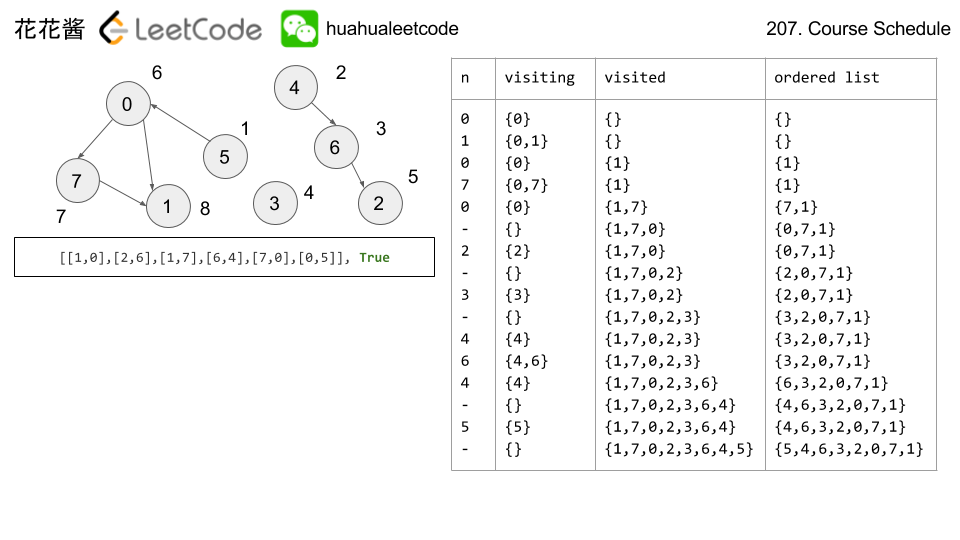# Solution 2: DFS Finding cycles

Time complexity: O(n^2)

Space complexity: O(n)

# Related Pr0blems:

Mission News Theme by Compete Themes.